Egwald Economics: Macroeconomics

by

Elmer G. Wiens

Egwald's popular web pages are provided without cost to users.
Please show your support by joining Egwald Web Services as a Facebook Fan:Follow Elmer Wiens on Twitter:Macroeconomics Theory, Testing, and Applications

The Basic Keynesian IS-LM Model

Open Economy Product Market and the IS(P) Curve

External Sector.

 Price level. System 1: price level P = 1. System 2: price level P = 1.03 [.85 - 1.15] Exchange rate. System 1: exchange rate ex = 0.67. System 2: exchange rate ex = 0.77 [.55 - 1.05].

Export Function.

The export function is a behavioural relation describing the foreign demand for domestically produced goods by firms. I hypothesize that these foreign purchases are a function of the domestic price level, P , and the exchange rate, ex.

xp = xp(P, ex)

xp = xp1 - xp2 * P - xp3 * ex

xp1, xp2, xp3 >= 0

Import Function.

The import function is a behavioural relation describing the demand for foreign produced goods by individuals, firms, and the government. I hypothesize that these purchases are a function of domestic prices, P, the exchange rate, ex, and domestic national income, y.

mp = mp(P, ex, y)

mp = mp1 + mp2 * P + mp3 * ex + mp4 * y

mp1, mp2, mp3, mp4 >= 0

The Net Export Function.

netxp = netxp(P, ex, y) = xp(P, ex) - mp(P, ex, y)

netxp = nxp1 - nxp2 * P - nxp3 * ex - nxp4 * y

nxp1, nxp2, nxp3, nxp4 >= 0

The estimates for the Net Export Function are based, partly, on regression estimates for the Canadian Economy over the years 1982-2000. Differentials in price level and exchange rate adjustments among countries affect exports and imports in a complex manner, preventing me from estimating simple, separate Export and Import Functions.

 System 1: netxp = 320 - 60 * P - 130 * ex - 0.15 * y System 2: netxp = 355 - 60 * P - 130 * ex - 0.15 * y Range 250 - 420 40 - 100 100 - 160 0.1 - 0.2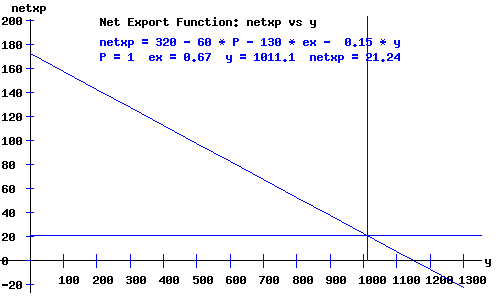Consumers (Individuals). The Consumer Expenditure Function. c = c(yd, r) c = c1 + c2*yd - c3*r + c4*r2 c1, c2, c3, c4 >= 0 c = 100 + 0.7*yd - 20*r + 0.5*r2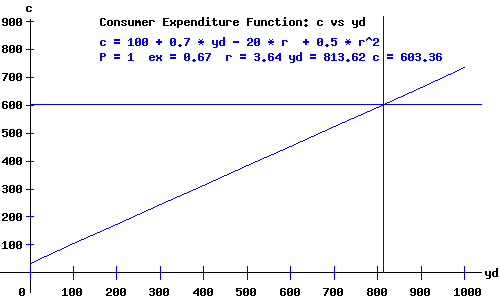Producers (Firms). The Private Firms' Investment Function. i = i(y, r) i = i1 + i2*y - i3*r + i4*r2 i1, i2, i3, i4 >= 0 i = 100 + 0.2*y - 40*r + 1.5*r2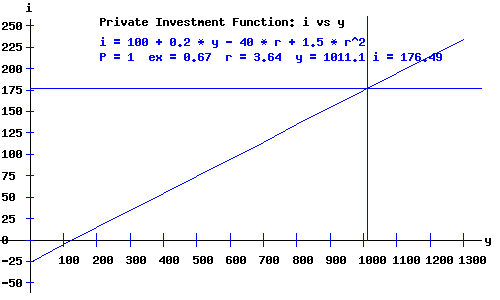The Government. Revenues and the Tax Function. t = t(y) t = -t1 + t2*y t1, t2 >= 0 t = -25 + 0.22*y.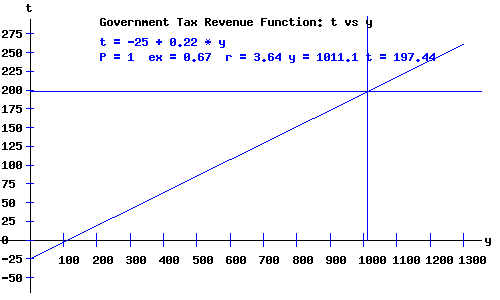Disposable Income Disposable national income, yd equals national income less taxes: System 1: yd = y - (-25 + 0.22*y) = 25 + 0.78*y = 25 + 0.78 * 1011.06 = 813.62. System 2: yd = y - (-25 + 0.22*y) = 25 + 0.78*y = 25 + 0.78 * 1021.92 = 822.1. Government Expenditures. In this basic macroeconomic model, I fixed government expenditures g = 210 billion dollars.

 The Product (Commodity) Market Equilibrium. The commodity markets equilibrate the demand and supply for exports and imports, consumer goods and services, investment goods, and goods and services purchased by the government. I aggregate the value of these products into one generic good. I assume that the value of the supply of products produced (and hence national income) will adjust to meet the value of the demand for products —> aggregate supply, AS = aggregate demand, AD. The product market equilibrium occurs at the level of national income where AD = AS, i.e., the level of income y where c + i + g + netxp = y. The diagram below depicts the equilibrium where c + i + g + netxp crosses the line (in yellow) with a 45 degree angle with the y-axis.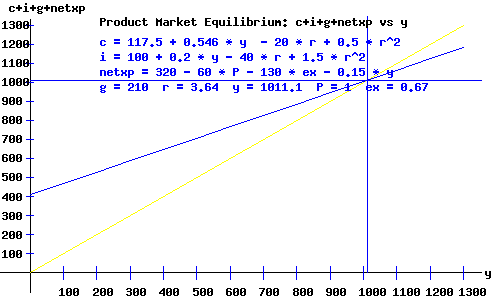The c + i + g + netxp curve as a function of y and r is: System 1: c + i + g + netxp = 600.4 + 0.596*y - 60*r + 2*r2 System 2: c + i + g + netxp = 620.6 + 0.596*y - 60*r + 2*r2 The IS(P) Curve. With the price level (GDP deflator) and the exchange rate as part of the model through the net export function, the location of the equilibrium in the product market depends on the values of P and ex The locus of interest rates and national incomes implicit for equilibrium in the commodity (product) market, an inverse relation between the rate of interest and national income, is called the IS(P) curve. The IS(P) curve, y as a function of the rate of interest r, is: System 1: y = 1486.14 - 148.51 * r + 4.95 * r2 System 2: y = 1536.14 - 148.51 * r + 4.95 * r2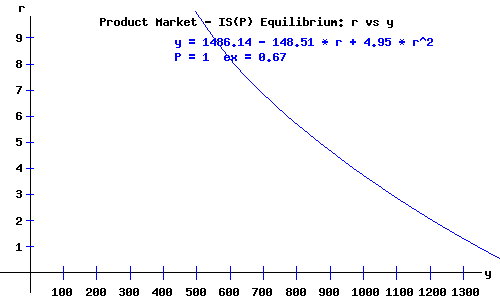Open Economy Money Market and the LM(P) Curve

 The Money Demand Function (Consumers and Producers). md = md(y, r) md = m1 + m2*y - m3*r + m4*r2 m1, m2, m3, m4 >= 0 md = 75 + 0.23*y - 35*r + 1.5*r2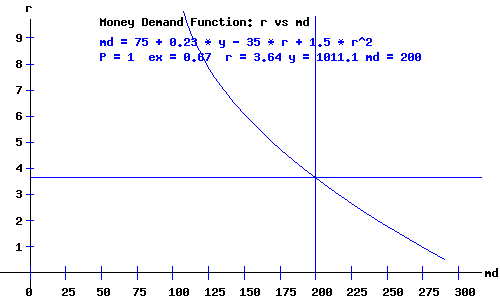Money Supply. I fixed the money supply ms = 200 billion dollars. With the price level (GDP deflator) as part of the model, the real supply of money, ms, equals the nominal supply of money, Ms, divided by the price level, P. System 1: With a price level of P = 1, and a nominal money supply of Ms = 200, the real money supply is: ms = Ms / P = 200. System 2: With a price level of P = 1.03, and a nominal money supply of Ms = 200, the real money supply is: ms = Ms / P = 194.17.

 The Money Market Equilibrium. System 1: With a price level of P = 1, a nominal money supply of Ms = 200, and national income of y = 1011.1, the equilibrium equation is: 200 = 307.54 - 35*r + 1.5*r2 with an equilibrium rate of interest r = 3.64. System 2: With a price level of P = 1.03, a nominal money supply of Ms = 200, and national income of y = 1021.9, the equilibrium equation is: 194.17 = 310.04 - 35*r + 1.5*r2 with an equilibrium rate of interest r = 3.99.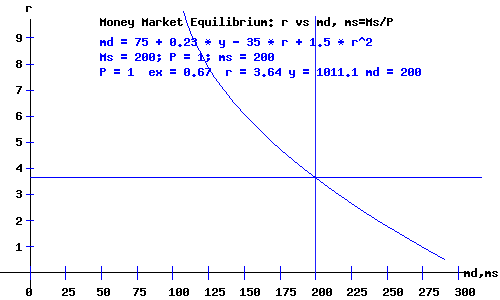The LM(P) Curve. I relabel the LM curve as LM(P) to indicate that it also depends on the price level. The equation of the LM(P) curve, y as a function of the rate of interest, r, and the price level, P, is: y = (Ms/P - m1)/m2 + m3/m2 * r - m4/m2 * r2; System 1: For a price of P = 1, and nominal money supply Ms = 200: The LM(P) curve, y as a function of the rate of interest r, is: y = 543.48 + 152.17 * r - 6.52 * r2 System 2: For a price of P = 1.03, and nominal money supply Ms = 200: The LM(P) curve, y as a function of the rate of interest r, is: y = 518.15 + 152.17 * r - 6.52 * r2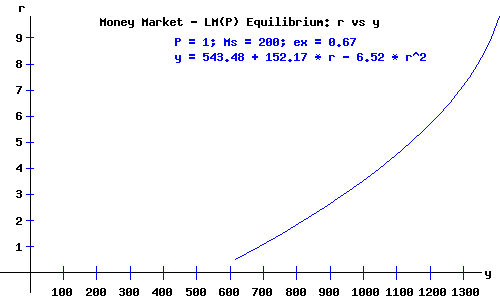The IS(P)-LM(P) Demand Equilibrium.

The IS(P)-LM(P) economy is in equilibrium when national income, y, the rate of interest, r, the price level, P, and the exchange rate, ex, are at levels consistent with equilibrium in both the product and money markets.

Economy equilibrium occurs where the IS(P) curve and the LM(P) curve intersect.

As a review, I list the system of equations for the IS(P)-LM(P) model. Solving these equations simultaneously, I obtain the values of the endogenous macroeconomics variables of the model: the amount of output and national income, y, and the rate of interest, r.

 Product Market - IS(P) Demand Equilibrium Consumers c = 100 + 0.7*yd - 20*r + 0.5*r2 Producers i = 100 + 0.2*y - 40*r + 1.5*r2 External Sector System 1: netxp = 320 - 60 * P - 130 * ex - 0.15 * y           System 2: netxp = 355 - 60 * P - 130 * ex - 0.15 * y Government Expenditures g = 210 Government Revenues t = -25 + 0.22*y Consumer Disposable Income yd = y - (-25 + 0.22*y) IS(P) Curve c + i + g + netxp = y Money Market - LM(P) Demand Equilibrium Consumers & Producers md = 75 + 0.23*y - 35*r + 1.5*r2 Nominal Money Supply; Real Money Supply Ms = 200 ; ms = Ms / P LM(P) Curve md = ms

For this basic model of the Canadian economy, the equilibrium obtains where:

System 1:

y = 1011.06,     r = 3.64 ,     P = 1 ,     ex = 0.67

System 2:

y = 1021.92,     r = 3.99 ,     P = 1.03 ,     ex = 0.77

The diagram below depicts the IS(P)-LM(P) economy demand equilibrium.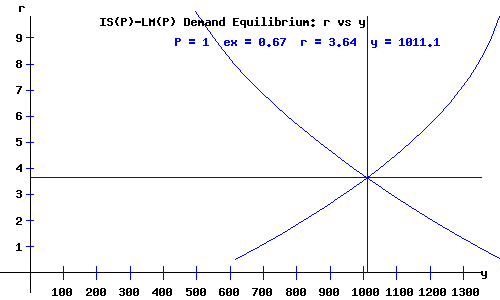Economy Equilibrium Macroeconomic Variables and Aggregates

 Blue System 1 Red System 2 National Income: y 1011.1 1021.9 Rate of Interest: r 3.64 3.99 Price Level: P 1 1.03 Exchange Rate: ex 0.67 0.77 Net Exports: netxp 21.24 39.81 Disposable National Income: yd 813.62 822.1 Consumer Expenditures: c 603.35 603.56 Firm Investments: i 176.46 168.55 Government Expenditures: g 210 210 Government Revenue: t 197.43 199.82 Nominal Money Supply: Ms 200 200 Real Money Supply: ms 200 194.17 Consumer Savings: s = yd - c 210.27 218.54

Works Cited and Consulted

• Akerlof, George A. "The Missing Motivation in Macroeconomics." Presidential Address, AEA, January, 2007.
• Branson, William H. and James M. Litvack. Macroeconomics. New York: Harper, 1976.
• Crouch, Robert L. Macroeconomics. New York: Harcourt, 1972.
• Darby, Michael. Macroeconomics. New York: McGraw-Hill, 1976.
• Dornbusch, Rudiger. Open Economy Macroeconomics. New York: Basic, 1980.
• Dornbusch, Rudiger, Stanley Fischer, and Gordon Sparks. Macroeconomics, 1st. Canadian Edition. Toronto: McGraw-Hill, 1982.
• Laidler, David E. W. The Demand For Money: Theories and Evidence. Scranton, Penn.: International Textbook, 1969.
• Parkin, Michael, and Robin Bade. Macroeconomics. 4th ed. Toronto: Addison Wesley, 2000.Copyright © Elmer G. Wiens:   EgwaldWeb ServicesAll Rights Reserved.    Inquiries